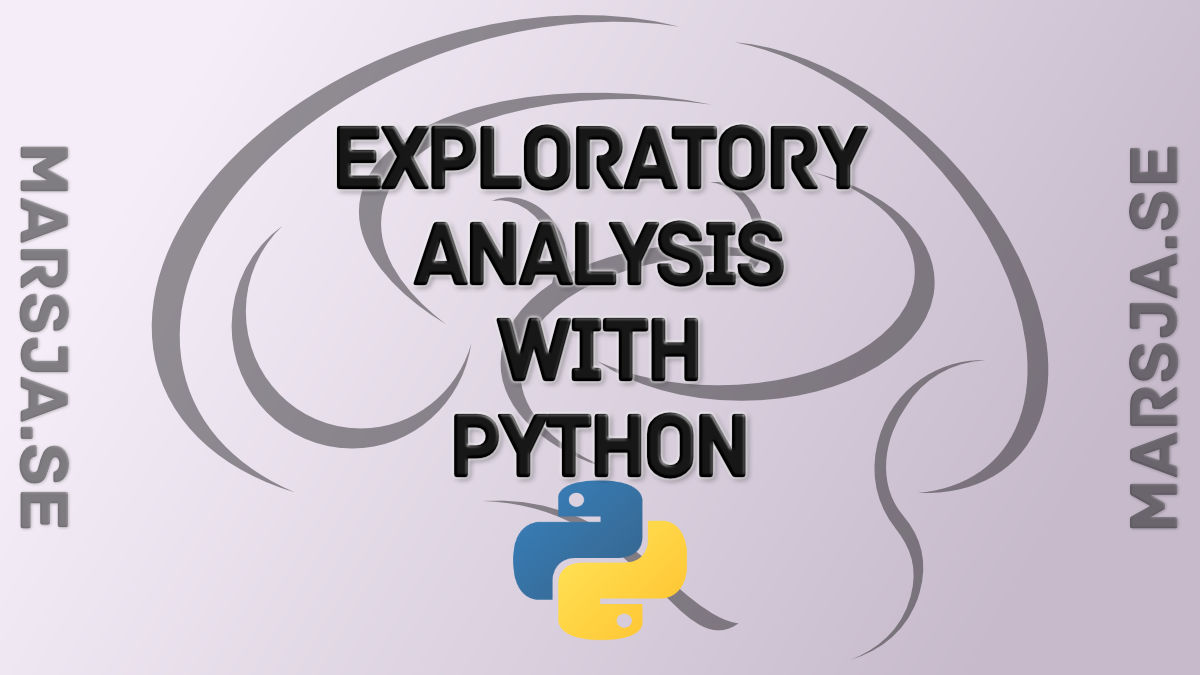## Exploratory Data Analysis in Python Using Pandas, SciPy, and Seaborn

In this post, we are going to learn how to explore data using Python, Pandas, and Seaborn. The data we are going to explore is data from a Wikipedia article. In this post, we are actually going to learn how to parse data from a URL using Python Pandas. Furthermore, we...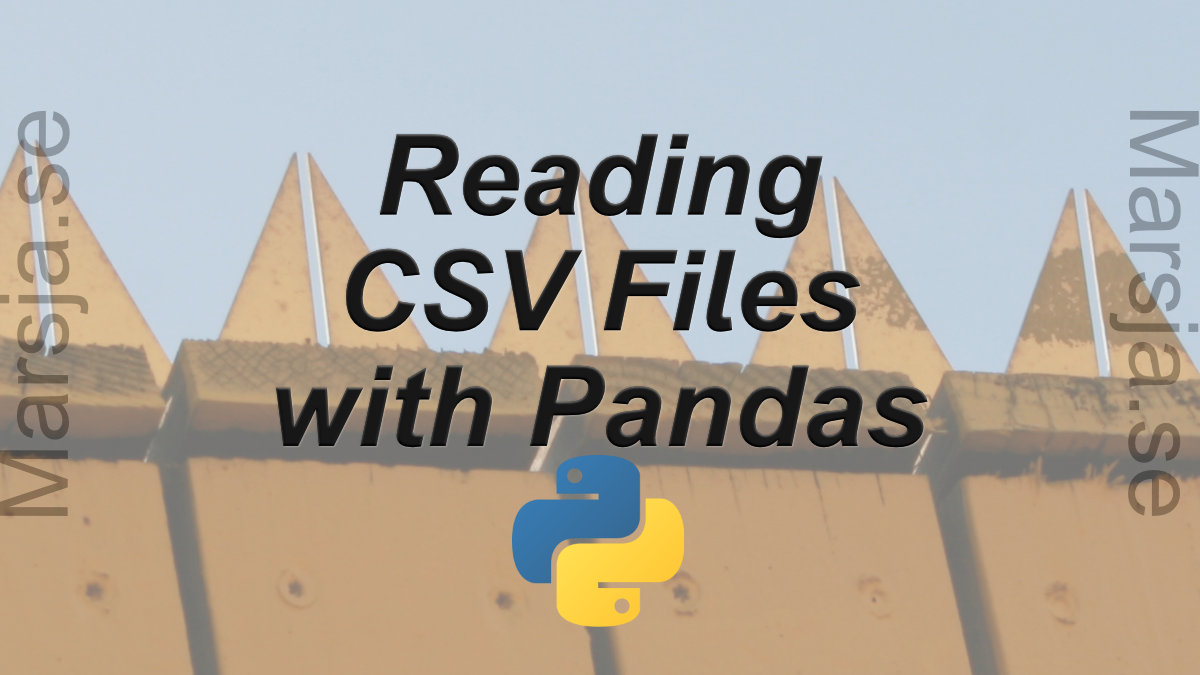In the first section, we will go through, with examples, how to read a CSV file, how to read specific columns from a CSV, how to read multiple CSV files and combine them to one dataframe, and, finally, how to convert data according to specific datatypes (e.g., using...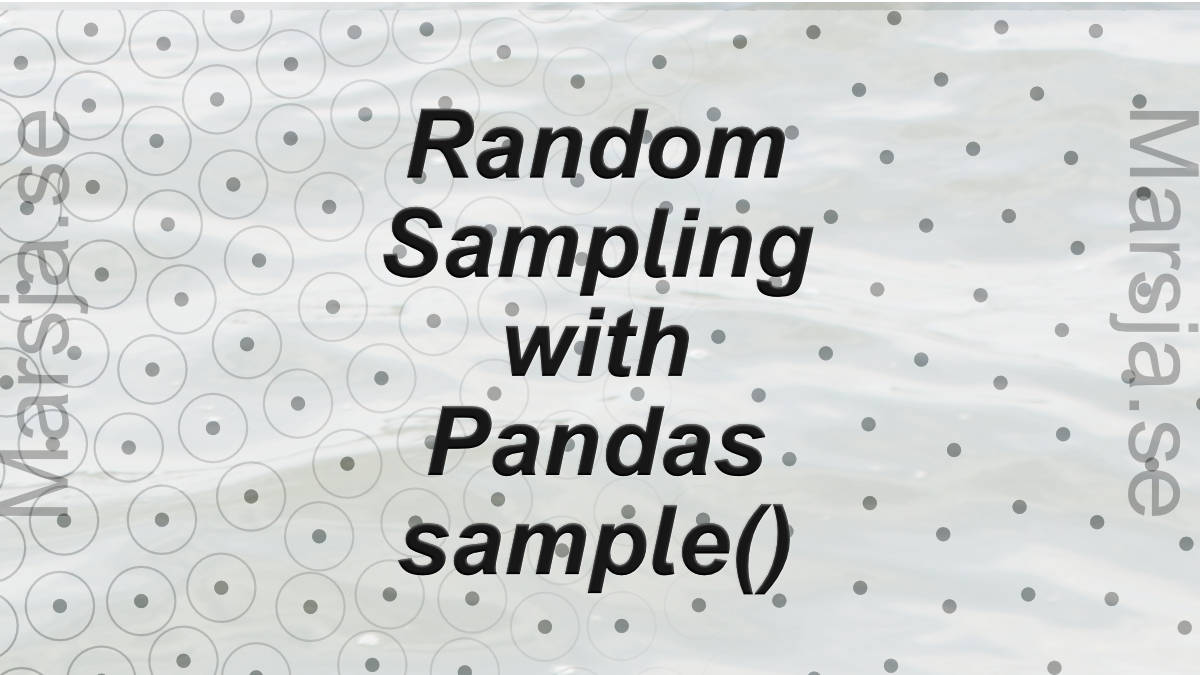## How to use Pandas Sample to Select Rows and Columns

In this tutorial, we will learn how to use Pandas sample to randomly select rows and columns from a Pandas dataframe. There are some reasons for randomly sample our data; for instance, we may have a very large dataset and want to build our models on a smaller sample...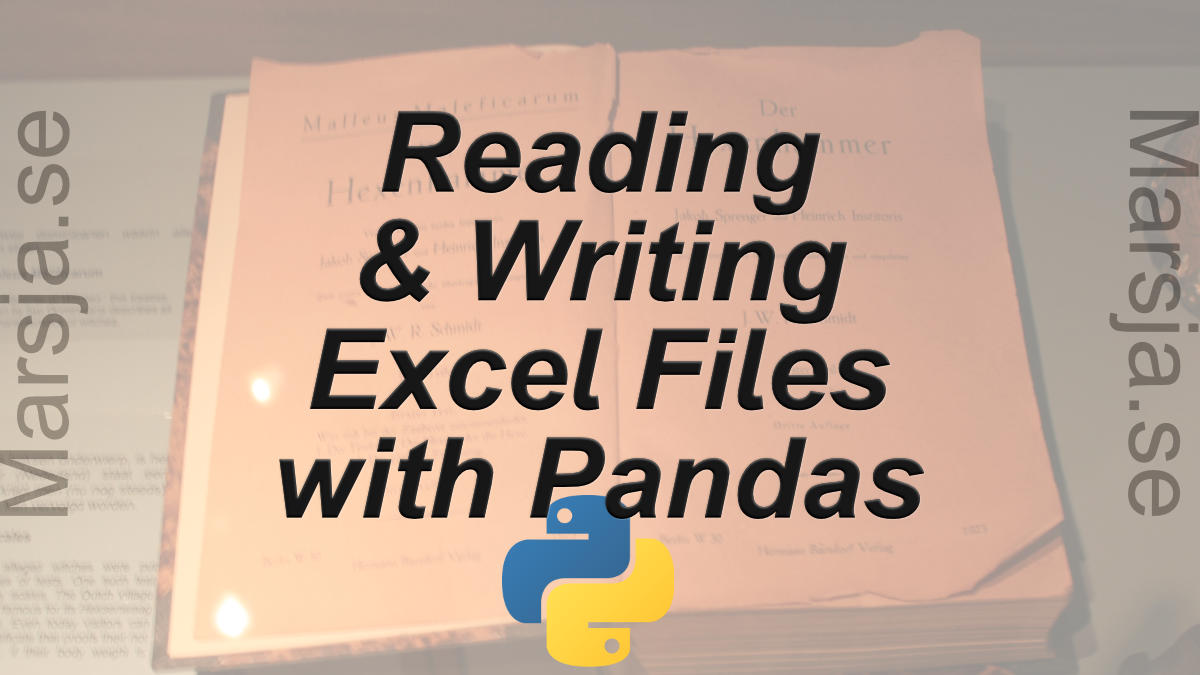## Pandas Excel Tutorial: How to Read and Write Excel files

In this Pandas Excel tutorial, we will learn how to work with Excel files in Python. It will provide an overview of how to use Pandas to load xlsx files and write spreadsheets to Excel. In the first section, we will go through, with examples, how to use Pandas...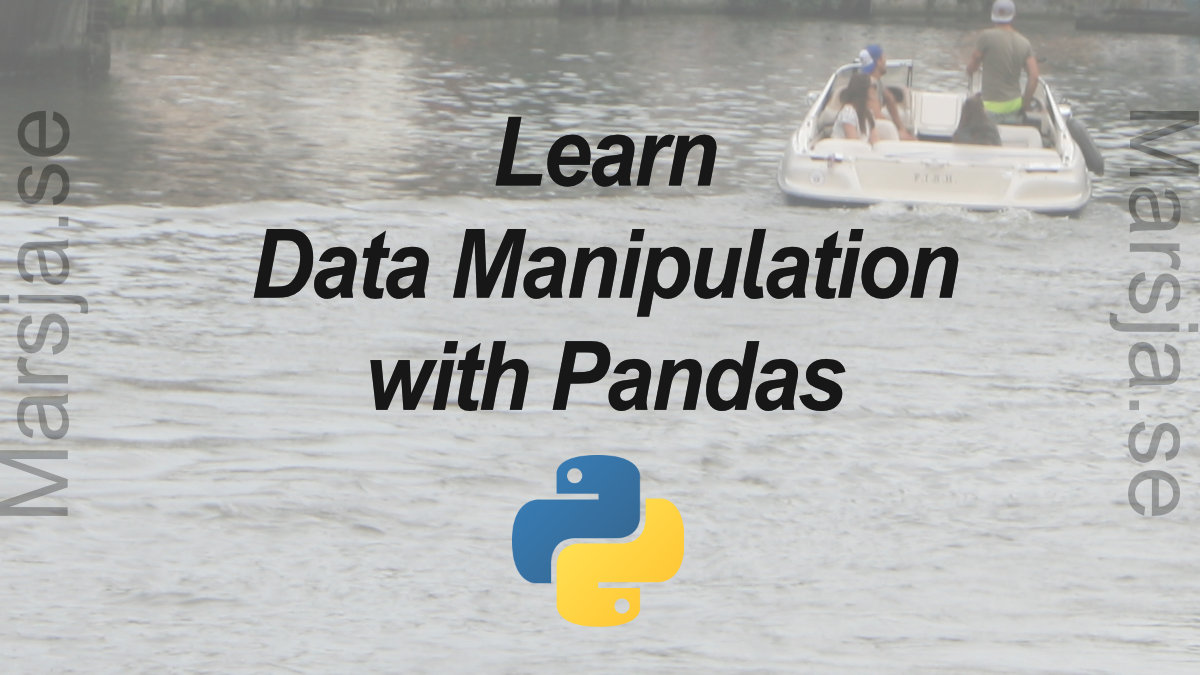## Data Manipulation with Pandas: A Brief Tutorial

Learn three data manipulation techniques with Pandas in this guest post by Harish Garg, a software developer and data analyst, and the author of Mastering Exploratory Analysis with pandas. Modifying a Pandas DataFrame Using the inplace Parameter In this section,...